kmp 订阅
KMP算法是一种改进的字符串匹配算法，由D.E.Knuth，J.H.Morris和V.R.Pratt提出的，因此人们称它为克努特—莫里斯—普拉特操作（简称KMP算法）。KMP算法的核心是利用匹配失败后的信息，尽量减少模式串与主串的匹配次数以达到快速匹配的目的。具体实现就是通过一个next()函数实现，函数本身包含了模式串的局部匹配信息。KMP算法的时间复杂度O(m+n)   。 展开全文KMP算法是一种改进的字符串匹配算法，由D.E.Knuth，J.H.Morris和V.R.Pratt提出的，因此人们称它为克努特—莫里斯—普拉特操作（简称KMP算法）。KMP算法的核心是利用匹配失败后的信息，尽量减少模式串与主串的匹配次数以达到快速匹配的目的。具体实现就是通过一个next()函数实现，函数本身包含了模式串的局部匹配信息。KMP算法的时间复杂度O(m+n)   。

O（m+n） 

The Knuth-Morris-Pratt Algorithm 

D.E.Knuth等 

KMP算法 

Brute-Force 

kmp算法简介
KMP算法是三位学者在 Brute-Force算法的基础上同时提出的模式匹配的改进算法。Brute- Force算法在模式串中有多个字符和主串中的若干个连续字符比较都相等,但最后一个字符比较不相等时,主串的比较位置需要回退。KMP算法在上述情况下,主串位置不需要回退,从而可以大大提高效率   。

• kmp算法 KMP算法是一种改进的字符串匹配算法，由D.E.Knuth，J.H.Morris和V.R.Pratt同时发现，因此人们称它为克努特——莫里斯——普拉特操作（简称KMP算法）。KMP算法的关键是利用匹配失败后的信息，尽量减少模式串...
• 该文章由本人转载，因需求而整理成文档形式。文档对KMP算法进行了十分详细的讲解。值得学习，十分受用！
• 主要介绍了如何通过Java代码实现KMP算法,文中通过示例代码介绍的非常详细，对大家的学习或者工作具有一定的参考学习价值,需要的朋友可以参考下
• 由 D.E.Knuth、J.H.Morris 和 V.R.Pratt 三人共同提出了一个改进的模式匹配算法，称为 KMP 算法。当某一位匹配失败时，可以根据已匹配的结果进行判断。当模式串中的第 k 位 与目标串的第 i 位比较发生不匹配时，...
•算法
• KMP模板 ========================================================================
• 给定一个子串，要求找出某个字符串中该子串的第一次出现的位置，即实现各种模式匹配。本资源中含有bf算法，和kmp算法，以及改进后的kmp算法
• 要搞懂kmp算法，首先要了解next数组 那么，next数组到底是求什么的呢？ 举个例子，有一个字符串abcabdabc, 要求它的最长的相同前缀后缀。 所谓前缀，就是包含了首字母的字符串字串； 所谓后缀，就是包含了末尾字母的...
• 第一个任务是要求用自己最擅长的语言编程读取一个TXT...KMP的预处理时间O（M），匹配时间复杂度O（N）；BM的预处理 O（N+M^2），匹配时间复杂度O（N）。因为所需处理的数据量不大，因此我选择用KMP算法来改进匹配效率。
• 如何用KMP字符串匹配算法求出主串中所包含模式串的总个数 #include using namespace std; void getnext(int next[],string s,int len) { int j=0,k=-1; next=-1; while(j<len){ if(k==-1||s[j]==s[k]){ j...
• 我们这里说的KMP不是拿来放电影的（虽然我很喜欢这个软件），而是一种算法。KMP算法是拿来处理字符串匹配的。今天我们谈到的是对KMP算法的拓展
• 主要介绍了C++ 数据结构之kmp算法中的求Next()函数的算法的相关资料,需要的朋友可以参考下
• 里面含有传统字符串匹配以及kmp算法匹配，包含kmp 导出的Archive file 文件修改jdk可以直接运行
• D-KMP体系结构-官方样本 这是D-KMP架构的官方示例，展示了一个适用于Android和iOS的简单主/详细应用程序。 有关D-KMP体系结构的更多信息，请阅读相关的。 D-KMP体系结构的主要功能： 它使用最新的声明性UI工具包：...Kotlin
• 本篇文章是对快速模式匹配算法(KMP)进行了详细的分析介绍，需要的朋友参考下
• 记录一下串里面的模式匹配，模式匹配，顾名思义就是给定一个被匹配的字符串，然后用一个字符串模式（模型）去匹配上面说的字符串，看后者是否在前者里面出现。常用的有2种算法可以实现，下面我们来具体探讨下
• kmp算法基础讲解，适于从零开始了解KMP算法的朋友。课程内容简单易懂。
• 一.BF算法  BF算法是普通的模式匹配算法，BF算法的思想就是将目标串S的第一个字符与模式串P的第一个字符进行匹配，若相等，则继续比较S的第二个字符和P的第二个字符；若不相等，则比较S的第二个字符和P的第一个字符...
• * Java实现KMP算法 * * 思想：每当一趟匹配过程中出现字符比较不等，不需要回溯i指针， * 而是利用已经得到的“部分匹配”的结果将模式向右“滑动”尽可能远 * 的一段距离后，继续进行比较。 * * 时间复杂度O...算法
• KMP算法是一种改进的字符串匹配算法，由D.E.Knuth，J.H.Morris和V.R.Pratt提出的，因此人们称它为克努特—莫里斯—普拉特操作（简称KMP算法）。KMP算法的核心是利用匹配失败后的信息，尽量减少模式串与主串的匹配...
• ## KMP

千次阅读 2019-11-22 11:36:00
KMP

最长公共子序列：https://blog.csdn.net/qq_40794973/article/details/102724108300. 最长上升子序列：https://blog.csdn.net/qq_40794973/article/details/102710180Algorithms：https://algs4.cs.princeton.edu/53substring/KMP.java.html

1 KMP 演示
1.1 求解最长公共前后缀

最长公共前后缀求解方法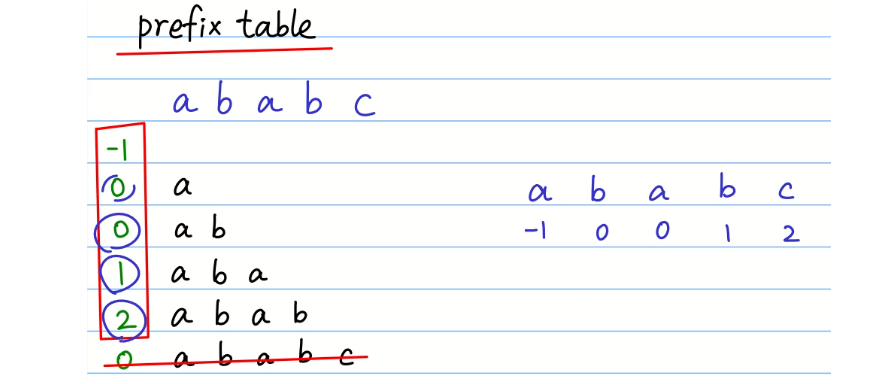1.2 匹配过程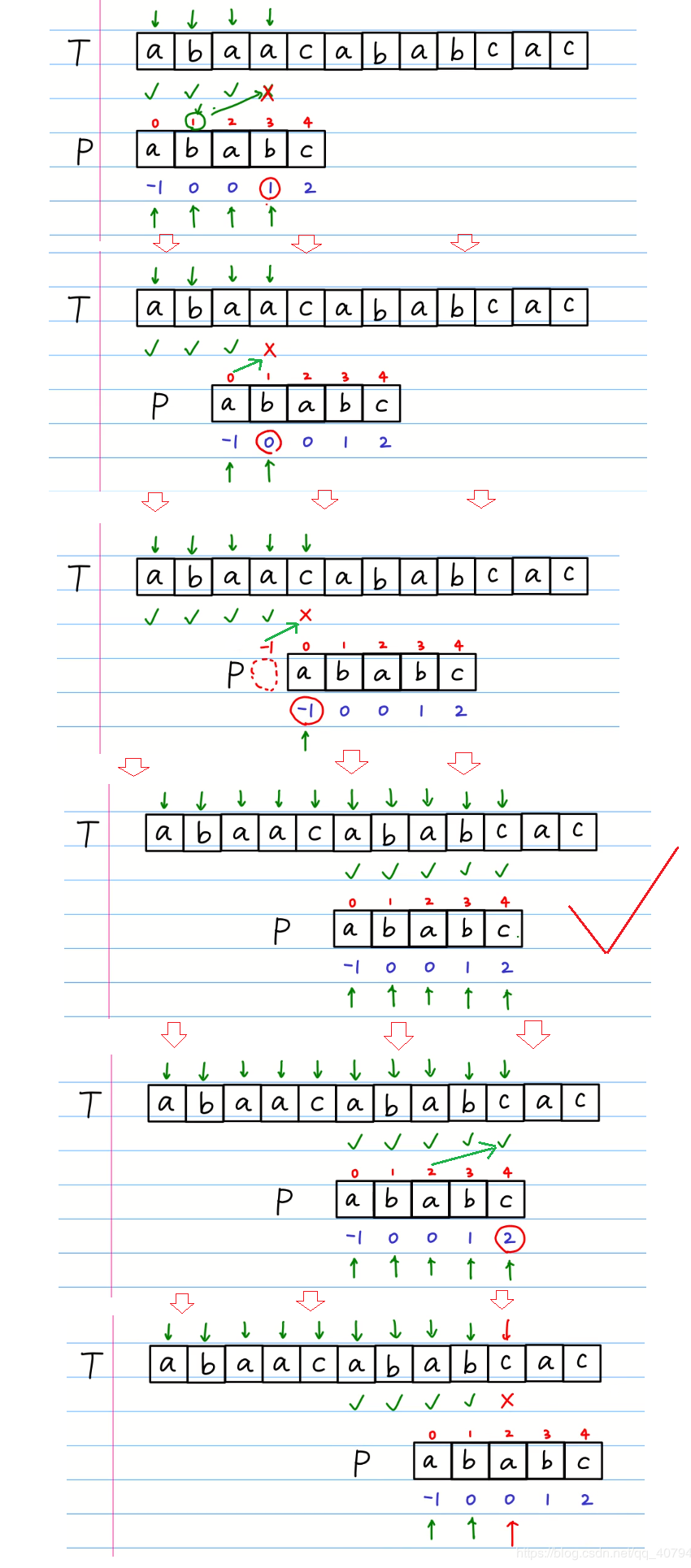2 KMP code
2.1 构建前缀表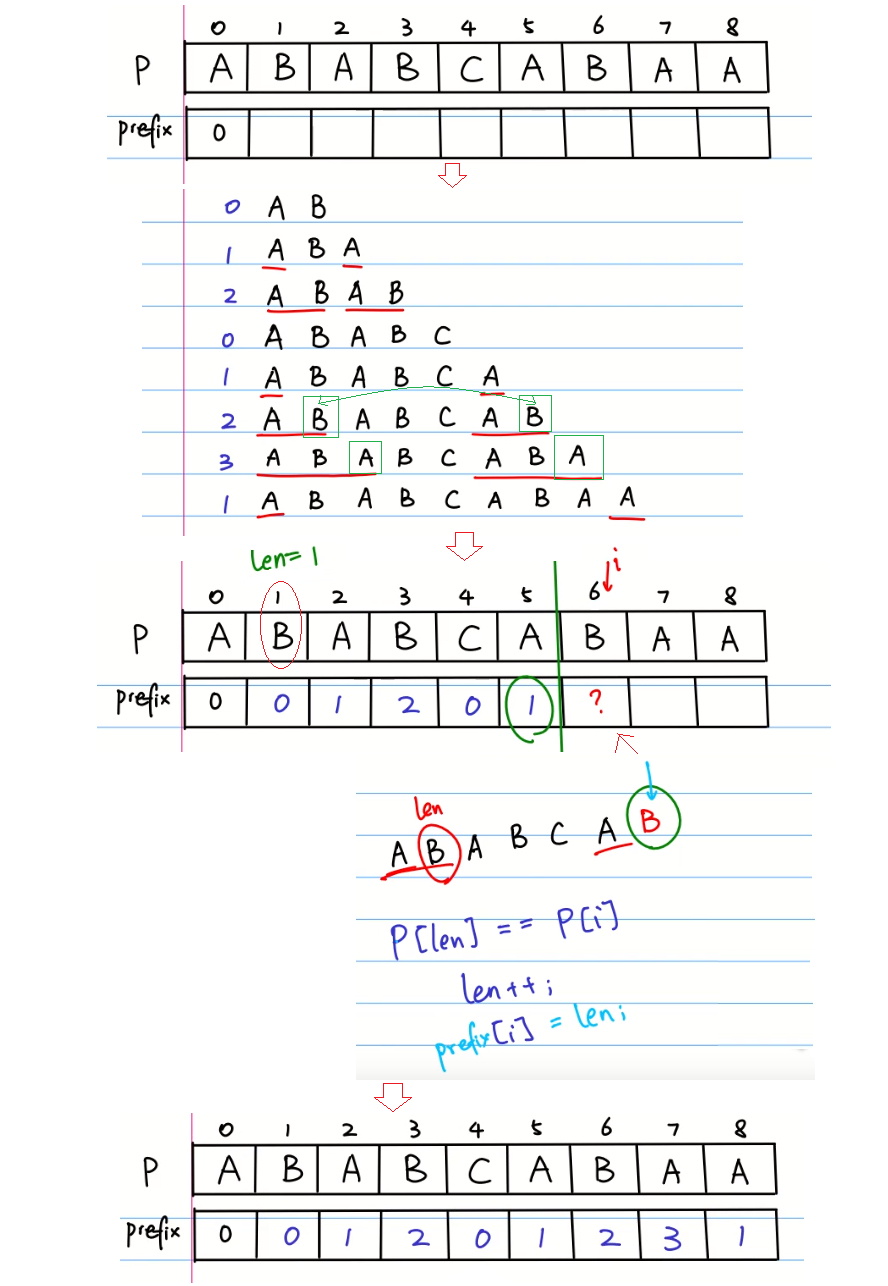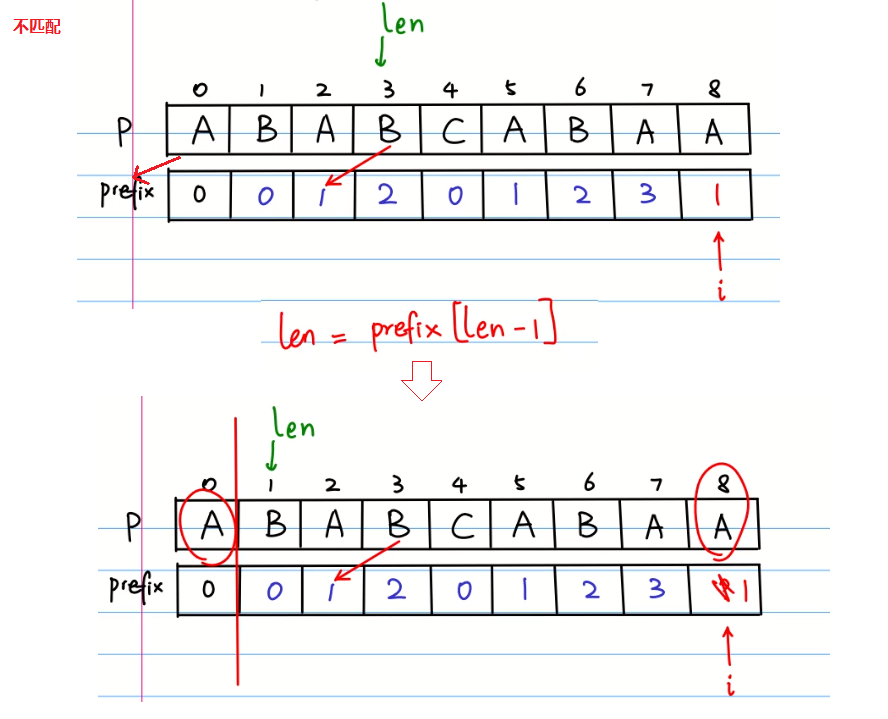/**
* 构建前缀表
* @param pattern 比较的文字
* @param prefix  prefix数组
*/
private static void prefixTable(char[] pattern, int[] prefix) {
int n = pattern.length;
prefix = 0;
int len = 0;
int i = 1;
while (i < n) {
if (pattern[i] == pattern[len]) {
len++;
prefix[i] = len;
i++;
} else {
if (len > 0) {
len = prefix[len - 1];
} else {//len == 0
prefix[i] = len;
i++;
}
}
}
}
/**
* 前缀表整体后移一位，第一位改成-1
*/
private static void movePrefixTable(int[] prefix) {
for (int i = prefix.length - 1; i > 0; i--) {
prefix[i] = prefix[i - 1];
}
prefix = -1;
}
//char[] pattern = "ABABCABAA".toCharArray();
//int[] perfix = new int[pattern.length];
System.out.println(perfix.length);
//prefixTable(pattern, perfix);
[0, 0, 1, 2, 0, 1, 2, 3, 1]
//System.out.println(Arrays.toString(perfix));
//movePrefixTable(perfix);
[-1, 0, 0, 1, 2, 0, 1, 2, 3]
//System.out.println(Arrays.toString(perfix));
2.2 开始匹配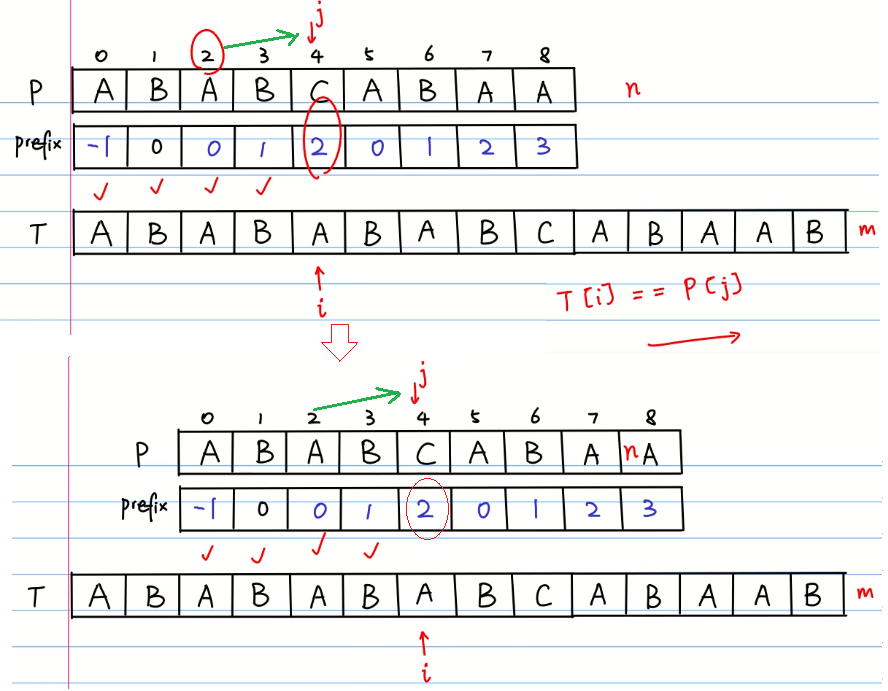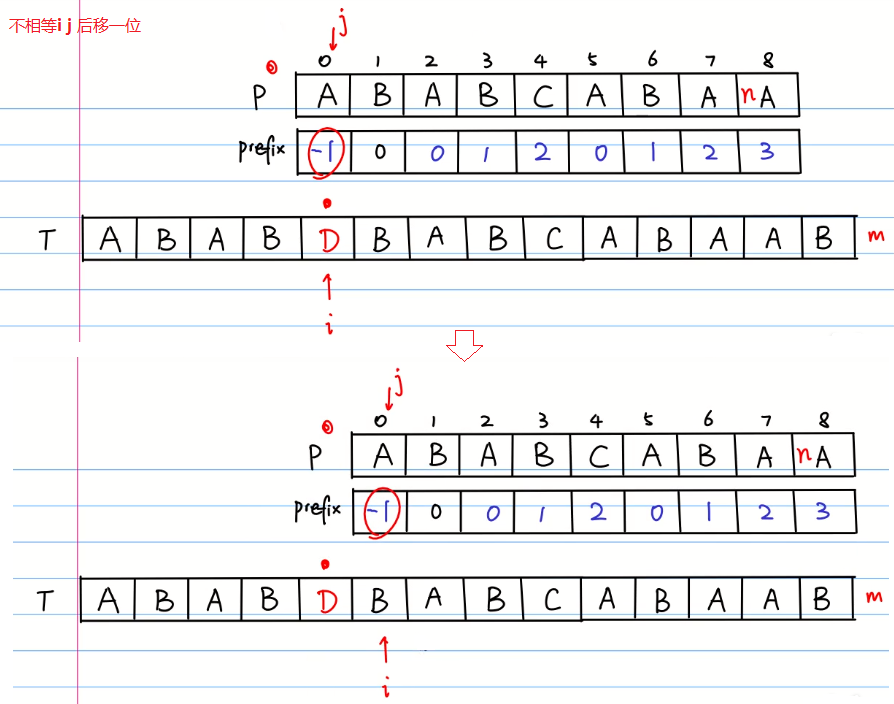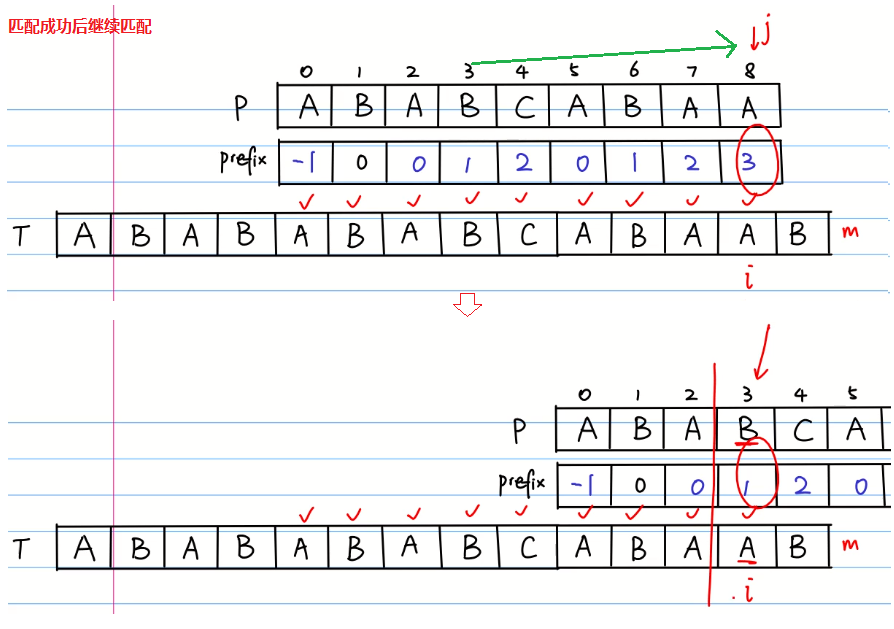public static void kmpSearch(char[] text, char[] pattern) {
int[] perfix = new int[pattern.length];
prefixTable(pattern, perfix);
movePrefixTable(perfix);
// text[i]    , text.length    = m
// pattern[j] , pattern.length = n
int i = 0;
int j = 0;
int m = text.length;
int n = pattern.length;
while (i < m) {
if (j == n - 1 && text[i] == pattern[j]) {
System.out.println("Found pattern at " + (i - j));
j = perfix[j];
}
if (text[i] == pattern[j]) {
i++;
j++;
} else {
j = perfix[j];
if (j == -1) {
i++;
j++;
}
}
}
}
//char[] pattern = "ABABCABAA".toCharArray();
//char[] text = "ABABABCABAABABABAB".toCharArray();
Found pattern at 2
//kmpSearch(text, pattern);

3 其他实现
public class KMP {
private final int R;       // the radix
private int[][] dfa;       // the KMP automoton
private char[] pattern;    // either the character array for the pattern
/**
* Preprocesses the pattern string.
* @param pattern the pattern string
* @param R       the alphabet size
*/
public KMP(char[] pattern, int R) {
this.R = R;
this.pattern = new char[pattern.length];
for (int j = 0; j < pattern.length; j++) {
this.pattern[j] = pattern[j];
}
// build DFA from pattern
int m = pattern.length;
dfa = new int[R][m];
dfa[pattern] = 1;
for (int x = 0, j = 1; j < m; j++) {
for (int c = 0; c < R; c++) {
dfa[c][j] = dfa[c][x];     // Copy mismatch cases.
}
dfa[pattern[j]][j] = j + 1;      // Set match case.
x = dfa[pattern[j]][x];        // Update restart state.
}
}
/**
* Returns the index of the first occurrrence of the pattern string
* in the text string.
* @param text the text string
* @return the index of the first occurrence of the pattern string
* in the text string; N if no such match
*/
public int search(char[] text) {
// simulate operation of DFA on text
int m = pattern.length;
int n = text.length;
int i, j;
for (i = 0, j = 0; i < n && j < m; i++) {
j = dfa[text[i]][j];
}
if (j == m) {
return i - m; // found
}
}
//char[] pattern =  "abra".toCharArray();
//KMP kmp2 = new KMP(pattern, 256);
//int offset2 = kmp2.search(text);
6
//System.out.println(offset2);

public class KMP {
private final int R;       // the radix
private int[][] dfa;       // the KMP automoton
private String pat;        // or the pattern string
/**
* Preprocesses the pattern string.
* @param pat the pattern string
*/
public KMP(String pat) {
this.R = 256;
this.pat = pat;
// build DFA from pattern
int m = pat.length();
dfa = new int[R][m];
dfa[pat.charAt(0)] = 1;
for (int x = 0, j = 1; j < m; j++) {
for (int c = 0; c < R; c++) {
dfa[c][j] = dfa[c][x];     // Copy mismatch cases.
}
dfa[pat.charAt(j)][j] = j + 1;   // Set match case.
x = dfa[pat.charAt(j)][x];     // Update restart state.
}
}
/**
* Returns the index of the first occurrrence of the pattern string
* in the text string.
* @param txt the text string
* @return the index of the first occurrence of the pattern string
* in the text string; N if no such match
*/
public int search(String txt) {
// simulate operation of DFA on text
int m = pat.length();
int n = txt.length();
int i, j;
for (i = 0, j = 0; i < n && j < m; i++) {
j = dfa[txt.charAt(i)][j];
}
if (j == m) {// found
return i - m;
}
}
//String pat = "ll";
//String txt = "hello";
//KMP kmp1 = new KMP(pat);
//int offset1 = kmp1.search(txt);
2
//System.out.println(offset1);

/*  text:    abacadabrabracabracadabrabrabracad
*
*  pattern: rab
*
*  pattern: bcara
*
*
*/

https://www.bilibili.com/video/av11866460?from=search&seid=8239914984865812627https://www.bilibili.com/video/av16828557?from=search&seid=8239914984865812627

展开全文• 在复习数据结构课程的过程中 对kmp算法及next数组的求解过程进行了深度探索 内含具体代码 及求解next数组的详解 望对大家有所帮助
• KMP算法实现的C++代码，KMP算法实现的C++代码，KMP算法实现的C++代码
• KMP KMP算法解决的问题 字符串str1和str2，str1是否包含str2，如果包含返回str2在str1中开始的位置。 如何做到时间复杂度O(N)完成？ 思路： 首先判断两个字符串是否为空串，并且str2的长度是否小于str1的长度，因为...
• KMP算法就是解决了这个问题，所以速度变得更快速了。 它是这样子的： 用一个数组：next[] 求得失配时的位置，然后保存下来。 要说清楚KMP算法，可以从朴素的模式匹配算法说起。  朴素的模式匹配算法比较容易理解，...
•algorithm
• 下面的的KMP算法的解释步骤 1. 首先，字符串”BBC ABCDAB ABCDABCDABDE”的第一个字符与搜索词”ABCDABD”的第一个字符，进行比较。因为B与A不匹配，所以搜索词后移一位。 2. 因为B与A不匹配，搜索词再往后移。 3....
•代码...